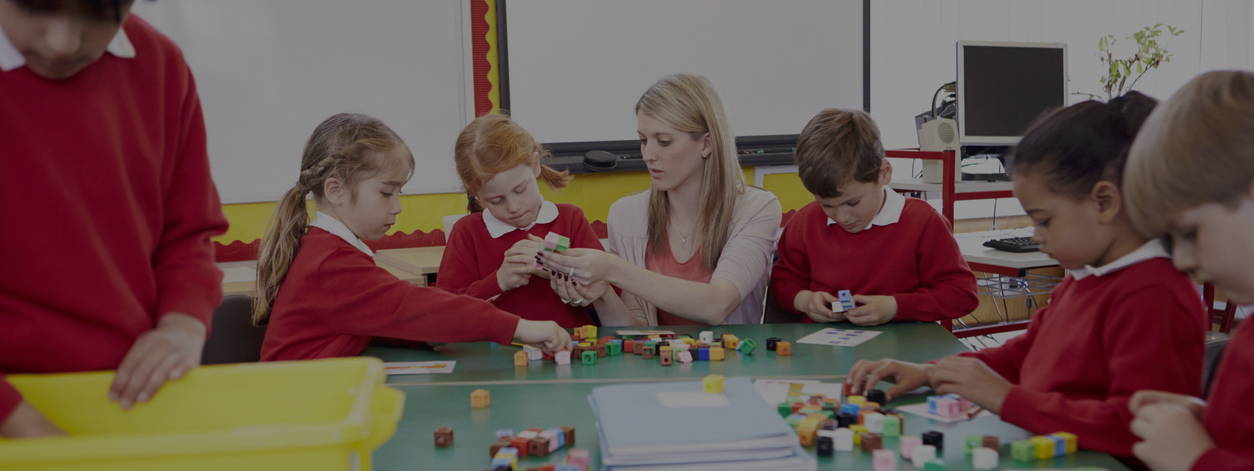# Addition and Subtraction Year 1

## Addition and Subtraction Year 1 Lesson Packs and FreeBees:

Year 1 Maths Lesson Pack

Let's Subtract Objects

Year 1 Maths Lesson Pack

Year 1 Maths Lesson Pack

Let's learn number bonds

Year 1 Maths Lesson Pack

Let's solve missing number problems

Year 1 Maths Lesson Pack

Let's use number facts

Year 1 Maths Lesson Pack

Number Bond Rainbows

Free KS1 Maths Activities

Number Bonds Worksheets

Free KS1 Maths Activities

# Addition and Subtraction Year 1

## What are the National Curriculum objectives for Year 1 Addition and Subtraction?

The National Curriculum 'Number - addition and subtraction' strand states that pupils in Year 1 should be taught to:

(–) and equals (=) signs
• represent and use number bonds and related subtraction facts within 20
• add and subtract one-digit and two-digit numbers to 20, including zero
• solve one-step problems that involve addition and subtraction, using concrete objects
and pictorial representations, and missing number problems such as 7 = ☐ – 9.

## Understanding number sentences and mathematical statements

Children in Year 1 begin to solve number sentences that are shared verbally before moving on to reading and interpreting written number sentences. Start by giving children practical word problems to help them develop an understanding of number sentences and mathematical symbols. For example:

There are two blue blocks and three red blocks on the table. How many blocks are there altogether? What does two plus three equal?

By providing the children with the objects as well as using a variety of mathematical language children can develop their maths skills, increase their knowledge of mathematical vocabulary and go at a pace that they feel comfortable with.

Vocabulary associated with addition and subtraction includes: add, addition, more, plus, make, sum total, altogether, subtract, subtraction, minus, leave, less, take away, and the difference between.

## Read, write and interpret mathematical statements involving addition (+) subtraction (-) and equals (=) signs

As children become familiar with the language associated with addition and subtraction they should start to read and write number sentences. Initially give children number sentences to read and solve, then as they become familiar with the symbols and structure of number sentences, challenge children to write and solve number sentences.

## Using concrete objects for addition and subtraction

A great place to start to help your Year 1 children understand addition and subtraction is to use concrete objects. This can be anything from things you have around the classroom, such as counters or cubes, to real-life objects such as apples, hairbands, pencils or stickers.

Use questions such as these while children are working with concrete objects:

• How many are there altogether?
• How many more do I need to add to make ten?
• If I take away seven objects, how many will be left?
• If you put together 4 pencils and 8 pencils, how many are there?
• What is three less than six?
• What is five more than two?

As children become more confident using concrete objects they can start to use objects to symbolically represent number sentences. This includes using cubes, unifix, numicon and dienes.

## Using pictorial representations for addition and subtraction

Once children are familiar with using concrete objects for addition and subtraction problems, they can move on to pictorial representations. These can be supported by concrete objects to help children relate the two.

For example, show children a plate with two biscuits and another plate with three biscuits with the number sentence written. Children can use the pictures that visually represent the number sentence to solve it. Once children are confident using this method, they can progress onto writing number sentences to correspond with pictorial representations as well as drawing pictures themselves to represent written number sentences.

As children become more confident with pictorial representations they can start to use marks and symbols to pictorially represent number sentences. This includes using ten frames.

## Using abstract representations for addition and subtraction

When children are able to confidently solve addition and subtraction problems using pictorial representations they should start to solve number sentences using mental methods. This requires children to use the skills of reading number sentences and working out the answer without using objects or pictures. They need to decode the information in the number sentence, figure out what the question is asking them to do and then solve it using their knowledge of numbers.